maths

Vector Algebra

Welcome to the only place where the essence of vectors is explained.

•  A vector in first-principles is a quantity with spatial-direction specified. Example: $2m$$2 m$ north-east.

•  A vector in component form is linear-combination of unit vectors of independent directions. eg: $2i+2j$$2 i + 2 j$

•  A vector in 3D co-ordinate system is a ray initiating from the origin.

Vector addition, dot product, and cross product are explained in

»  first principle,

»  geometrical meaning, and

»  component form.

The details explained here are revolutionary and astonishingly simple to understand.

maths > vector-algebra > vector-scalar-difference

Introducing Vectors

To completely specify and to use in mathematical calculations, some quantities require both magnitude and direction. calculations.

»  Measurement of Quantities
→  Addition: sum of measured amount
→  Subtraction: difference in measured amount

»  Some quantities do not add or subtract only in the amount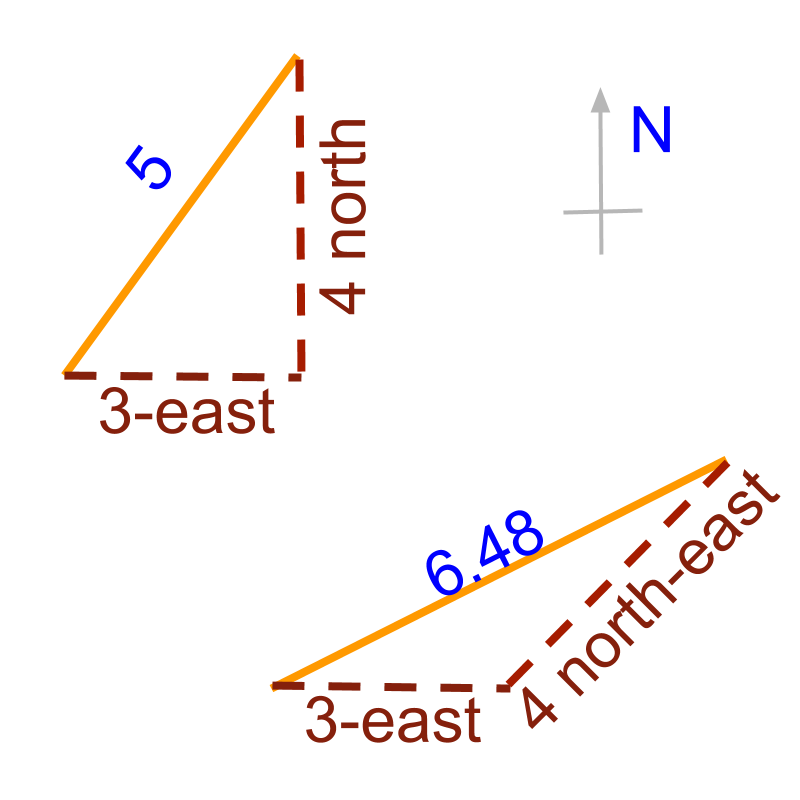→  $3$$3$ east + $4$$4$ north is not $7$$7$
→  $3$$3$ east + $4$$4$ north-east is approximately $6.48$$6.48$

»  Some quantities cannot be used in calculation if only amount is given without direction

»  Measurement of such quantities should capture both the amount and the direction to carryout calculations

maths > vector-algebra > representation-of-vectors

Mathematical Representation of Vectors

»  Vectors specify amount or magnitude and direction

→  $3$$3$m east and $4$$4$m north

→  $3$$3$m east and $4$$4$m north-east

»  How were the quantities added?

→  $3$$3$m east + $4$$4$m north = $\sqrt{{3}^{2}+{4}^{2}}$$\sqrt{{3}^{2} + {4}^{2}}$ as per Pythagoras theorem

→  $3$$3$m east + $4$$4$m north-east : Making this easy to do is the objective of "vector algebra"

»  Position of an airplane $ai+bj+ck$$a i + b j + c k$

→  3Okm east (x-axis) = $30i$$30 i$

→  40km north (y-axis) = $40j$$40 j$

→  4km altitude (z-axis) = 4k

»  component form of a vector $ai+bj+ck$$a i + b j + c k$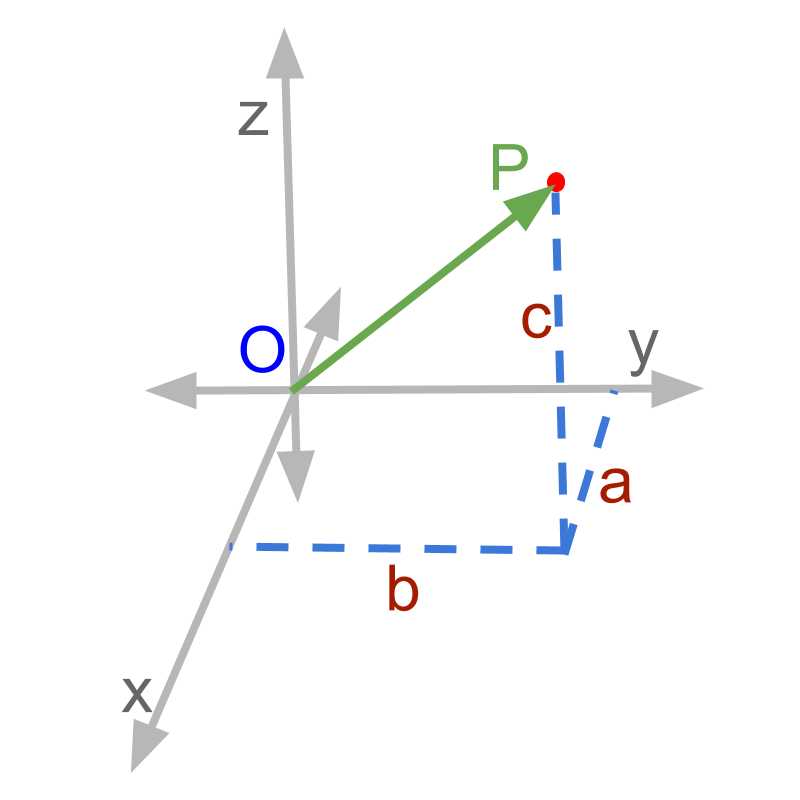→  $i$$i$,$j$$j$,$k$$k$ represent the direction along $x$$x$, $y$$y$, and $z$$z$ axes

→  $a$$a$, $b$$b$, $c$$c$ represent the amount or magnitude along the given directions

maths > vector-algebra > magnitude-of-vector

Magnitude of a Vector

In this page, computing magnitude of a vector is explained in detail. magnitude of $\stackrel{\to }{p}=ai+bj+ck$$\vec{p} = a i + b j + c k$ is $|\stackrel{\to }{p}|=\sqrt{{a}^{2}+{b}^{2}+{c}^{2}}$$| \vec{p} | = \sqrt{{a}^{2} + {b}^{2} + {c}^{2}}$→  length of $\overline{OP}$$\overline{O P}$ is the magnitude

maths > vector-algebra > types-of-vectors

Types of Vectors

Classification of Vectors

»  Classification by magnitude

→  null or zero vector : magnitude $0$$0$

→  unit vector : magnitude $1$$1$

→  proper vector : magnitude not $0$$0$<

»  By similarities of two vectors

→  equal vectors : All corresponding components equal

→  like vectors: same directions

→  unlike vectors : different directions

→  co-initial vectors : same initial point

→  co-linear vectors : on the same line

→  co-planar vectors : on the same plane

→  non-co-planar vectors : not on the same plane

maths > vector-algebra > properties-magnitude-of-vector

Properties of Magnitude of a Vector

In this topic, Properties of Magnitude of vectors are explained in detail.

»  Magnitude of null vectors $|\stackrel{\to }{0}|$$| \vec{0} |$$=0$$= 0$

»  Magnitude of a negative $|-\stackrel{\to }{p}|=|\stackrel{\to }{p}|$$| - \vec{p} | = | \vec{p} |$

»  Magnitude of an unit vector $|\stackrel{^}{p}|=1$$| \hat{p} | = 1$

maths > vector-algebra > relationship-between-vectors-coordinate-geometry

Vector and Coordinate Geometry

In this topic, you will learn about how vector algebra can be used in coordinate geometry and how coordinate geometry principles help in vector algebra problems.

»  Vector Algebra in Coordinate Geometry

→  Vector representation can be used to describe points in coordinate space.

→  $p\left(x,y,z\right)↔xi+yj+zk$$p \left(x , y , z\right) \leftrightarrow x i + y j + z k$

→  Distance between origin and the point is the magnitude of the vector.

Vector Algebra can be used to solve probems in coordinate geometry.

»  Coordinate Geometry in Vector Algebra

→  Vectors are directed line segments in coordinate space

→  Magnitude is the distance between initial and terminal points

→  Relative properties of vectors, like, angle between vectors, are preserved

Properties and results of coordinate geometry can be used to solve vector algebra problems

maths > vector-algebra > position-vector-of-a-point

Position Vector of a Point

In this page, you will learn about position vector of a point. This concept connects vector algebra and coordinate geometry.

»  For a point $P\left(a,b,c\right)$$P \left(a , b , c\right)$ the position vector is $\stackrel{\to }{OP}=ai+bj+ck$$\vec{O P} = a i + b j + c k$maths > vector-algebra > directional-cosine-of-a-vector

Directional Cosine

In this page, you will learn about the alternate representation of vectors other than $ai+bj+ck$$a i + b j + c k$?

Directional Cosine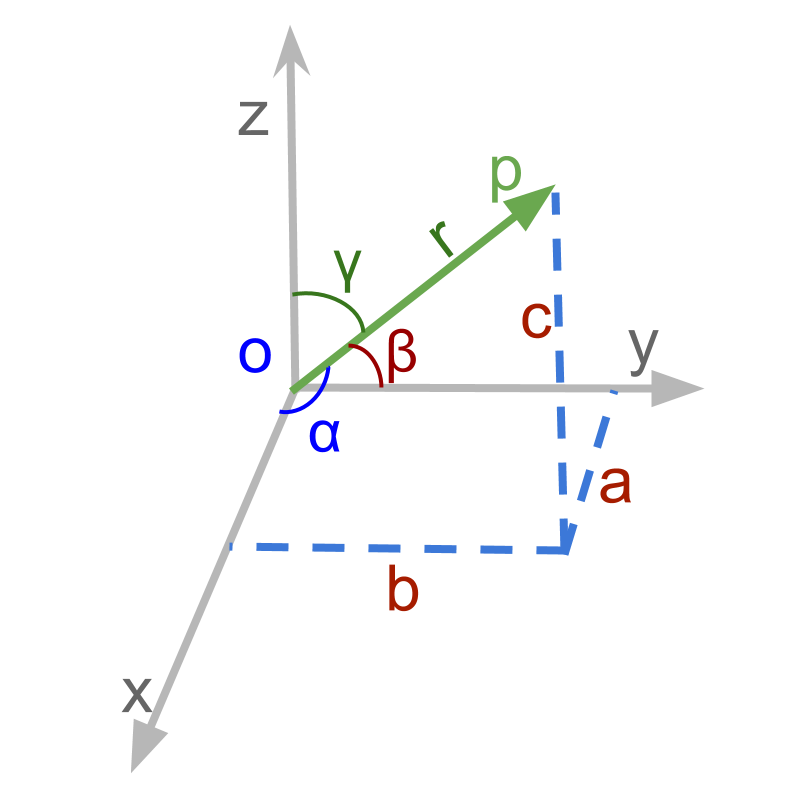→  $\stackrel{\to }{p}=ai+bj+ck$$\vec{p} = a i + b j + c k$ makes angles $\alpha ,\beta ,\gamma$$\alpha , \beta , \gamma$ with $x,y,z$$x , y , z$-axes respectively

→  $\mathrm{cos}\alpha =\frac{a}{|p|}$$\cos \alpha = \frac{a}{| p |}$

→  $\mathrm{cos}\beta =\frac{b}{|p|}$$\cos \beta = \frac{b}{| p |}$

→  $\mathrm{cos}\gamma =\frac{c}{|p|}$$\cos \gamma = \frac{c}{| p |}$

maths > vector-algebra > introduction-vector-arithmetic

Vector Arithmetic

In this page, the basic mathematical operations of vector algebra is introduced with examples. Vector Arithmetic

»  Application Scenarios of Vector Arithmetic

→  Subtraction : inverse of addition

→  Repeated addition of a quantity: a vector is multiplied by a scalar

→  Product between two quantities : two vectors can be multiplied

»  Two Possibilities of Vector Multiplication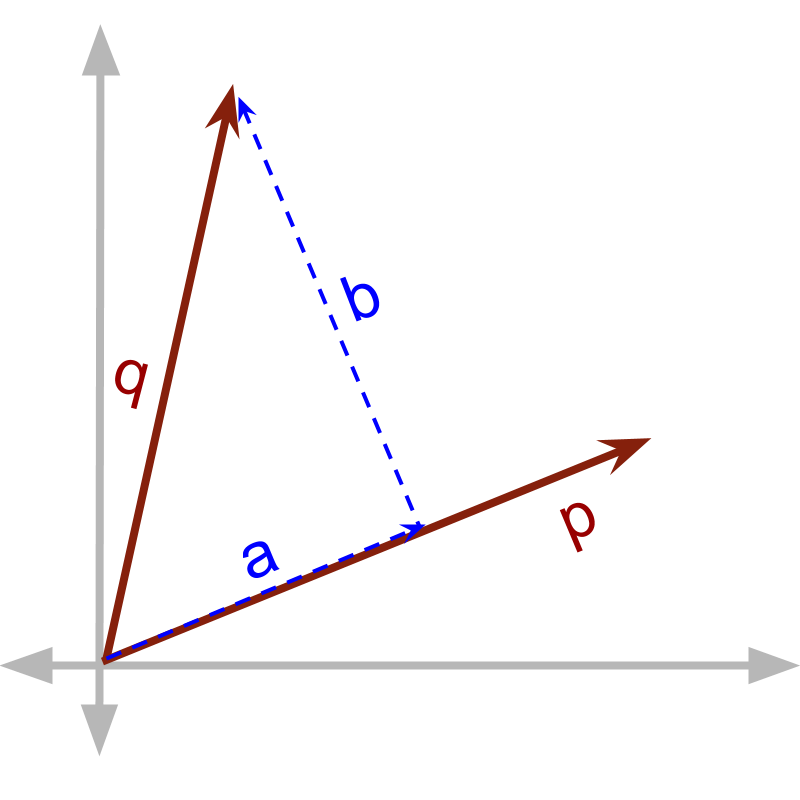→  Vector Dot Product : multiply with component in parallel

→  Vector Cross Product : multiply with component in perpendicular

»  Vector Division is not defined
→  Division is the inverse of multiplication. In vector multiplication, one of the components is lost in the product. And so vector division is not defined. All these arithmetic operations are explained in detail later. The objective of this is to understand the role of components in parallel and in perpendicular.

maths > vector-algebra > understanding-direction-of-vectors

Understanding Direction

How the property 'direction' affects vector quantities in mathematical calculations? Independence of Quantities along Orthogonal Directions

→  For a vector, a change along one axis affects only the component along that axis and do not affect the components along other axes, as the axes are orthogonal

»  Effect of Direction:

→  Addition involves right-triangles and Pythagorean identity

→  Product between quantities involves either one of component in parallel or perpendicular

»  Components in parallel add and component in perpendicular combine as right triangle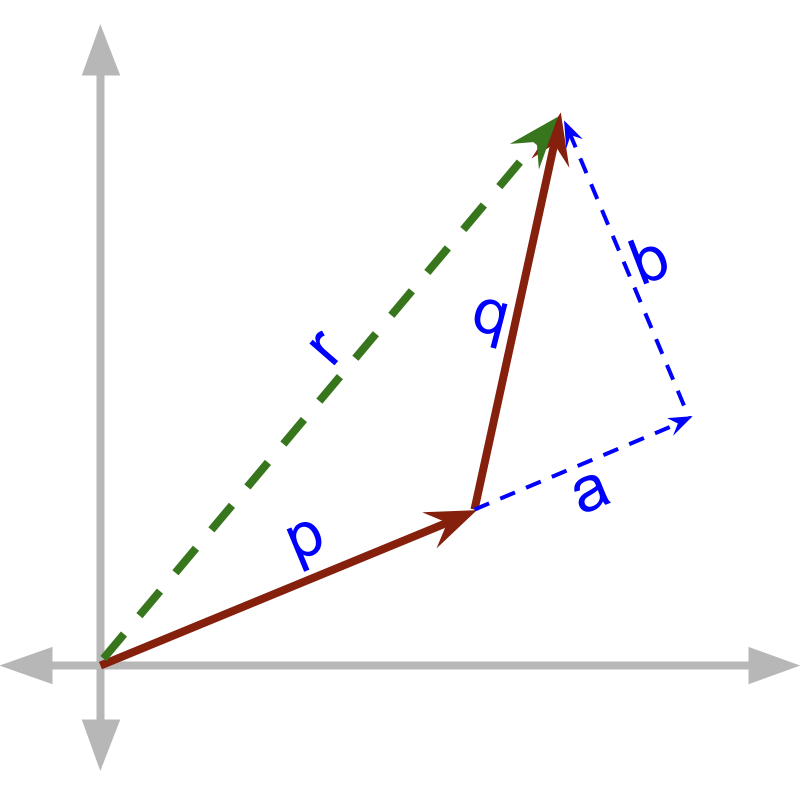→  component in parallel to $\stackrel{\to }{p}$$\vec{p}$ is $\stackrel{\to }{a}$$\vec{a}$
→  component in perpendicuar to $\stackrel{\to }{p}$$\vec{p}$ is $\stackrel{\to }{b}$$\vec{b}$
→  $\stackrel{\to }{p}+\stackrel{\to }{a}$$\vec{p} + \vec{a}$ and $\stackrel{\to }{b}$$\vec{b}$ result in $\stackrel{\to }{r}$$\vec{r}$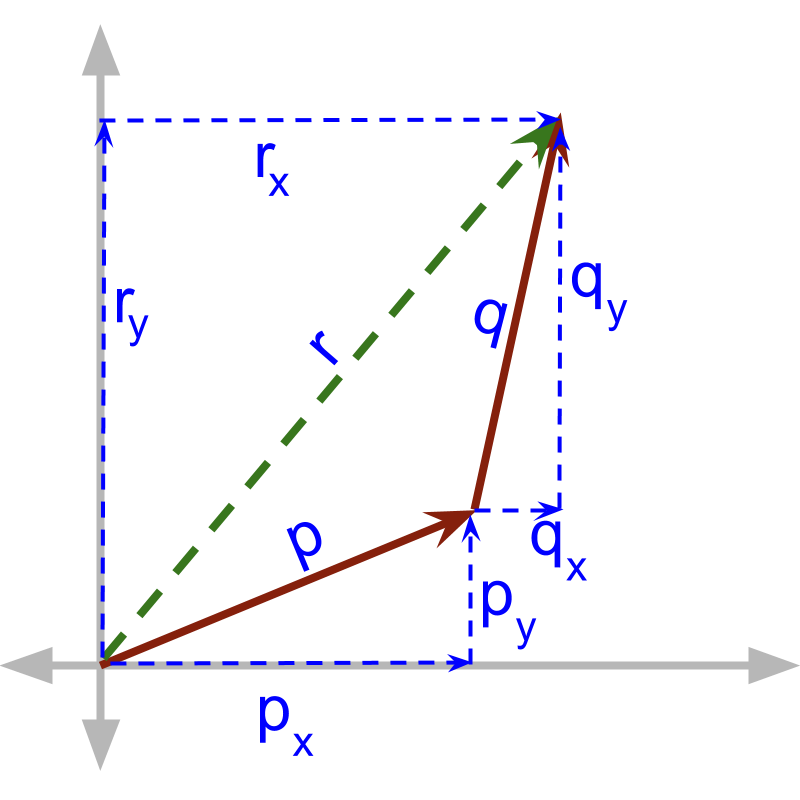→  ${r}_{x}={p}_{x}+{q}_{x}$${r}_{x} = {p}_{x} + {q}_{x}$
→  ${r}_{y}={p}_{y}+{q}_{y}$${r}_{y} = {p}_{y} + {q}_{y}$
→  ${r}_{z}={p}_{z}+{q}_{z}$${r}_{z} = {p}_{z} + {q}_{z}$

Triangular Law

sequential addition of $\stackrel{\to }{p}$$\vec{p}$ and $\stackrel{\to }{q}$$\vec{q}$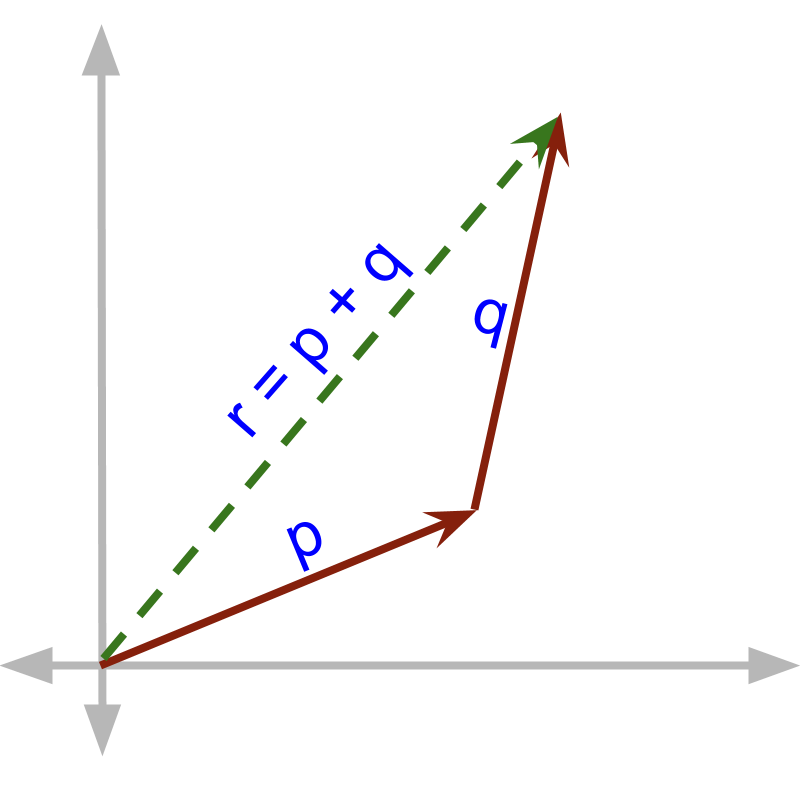→  the third side of the triangle formed by $\stackrel{\to }{p}$$\vec{p}$ and $\stackrel{\to }{q}$$\vec{q}$

Parallelogram Law

continuous addition of $\stackrel{\to }{x}$$\vec{x}$ and $\stackrel{\to }{y}$$\vec{y}$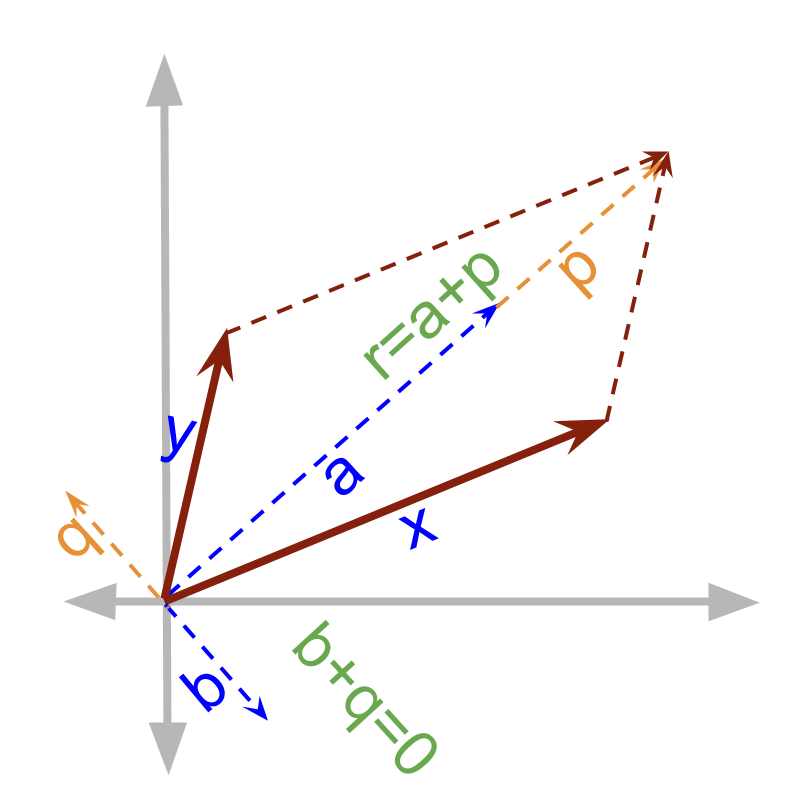→  $\stackrel{\to }{x}=\stackrel{\to }{a}+\stackrel{\to }{b}$$\vec{x} = \vec{a} + \vec{b}$

→  $\stackrel{\to }{y}=\stackrel{\to }{p}+\stackrel{\to }{q}$$\vec{y} = \vec{p} + \vec{q}$

→  the co-initial diagonal of the parallelogram formed by sides $\stackrel{\to }{x}$$\vec{x}$ and $\stackrel{\to }{y}$$\vec{y}$

»  Repeated addition is generalized to Multiplication of a vector by Scalar

»  Components are multiplied by scalar
→  $\stackrel{\to }{p}=ai+bj+ck$$\vec{p} = a i + b j + c k$
→  $\lambda \stackrel{\to }{p}=\lambda ai+\lambda bj+\lambda ck$$\lambda \vec{p} = \lambda a i + \lambda b j + \lambda c k$

maths > vector-algebra > standard-unit-vectors

Standard Unit Vectors

In this page, orthogonal axes of vector space and the standard unit vectors are explained.

»  $3$$3$ orthogonal axes are represented with $3$$3$ unit vectors
→  x-axis $i$$i$
→  y-axis $j$$j$
→  z-axis $k$$k$

maths > vector-algebra > vector-as-sum-of-vectors

Vector as sum of vectors

In this page, you will learn that Vectors can be represented as sum of several vectors.

»  Vectors as Linear combination of vectors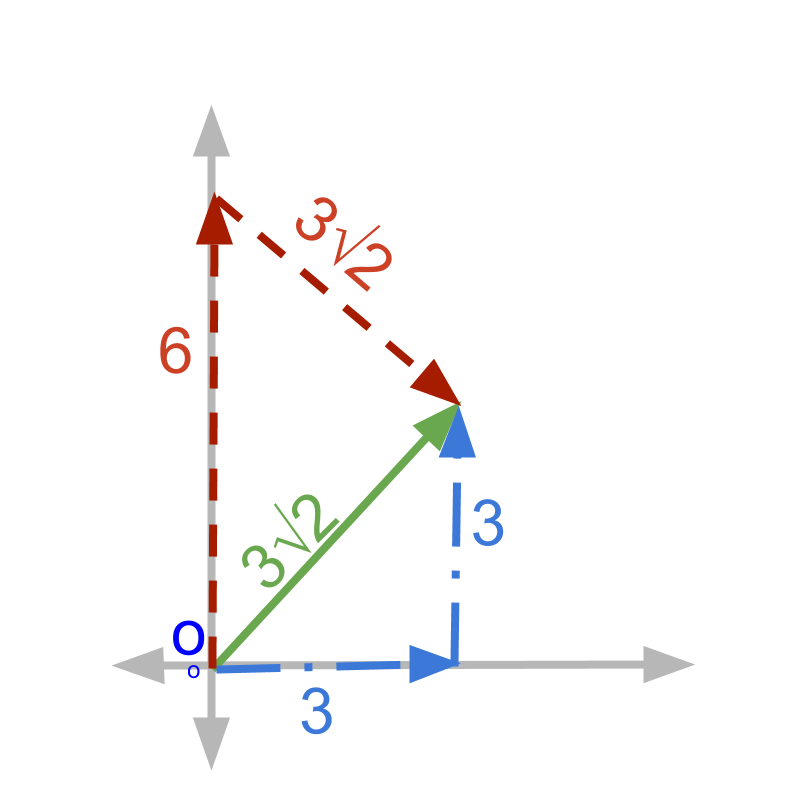→  $3\sqrt{2}$$3 \sqrt{2}$ at ${45}^{\circ }$${45}^{\circ}$ angle
→  $3$$3$ at ${0}^{\circ }$${0}^{\circ}$ + $3$$3$ at ${90}^{\circ }$${90}^{\circ}$
→  $6$$6$ at ${90}^{\circ }$${90}^{\circ}$ + $3\sqrt{2}$$3 \sqrt{2}$ at $-{45}^{\circ }$$- {45}^{\circ}$
All these represent the same vector.

maths > vector-algebra > vector-component-form

Component form of Vectors

Vectors are represented in component form as sum of standard unit vectors multiplied by scalars.

Vector Component Form is linear combination of vectors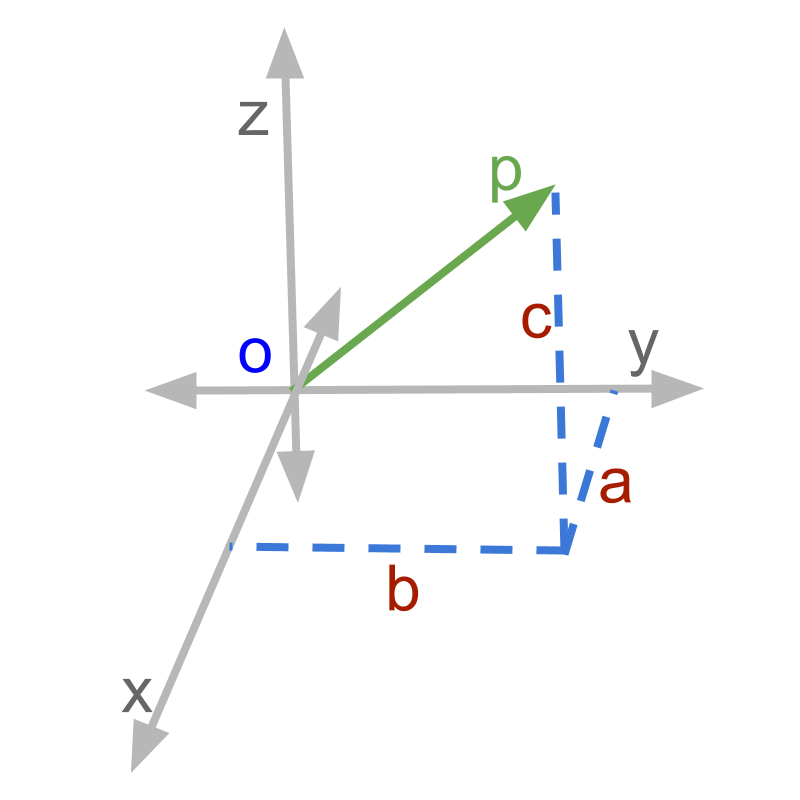→  $i$$i$, $j$$j$, $k$$k$ are unit vectors
→  $a$$a$, $b$$b$, $c$$c$ are scalars
→  $\stackrel{\to }{p}=ai+bj+ck$$\vec{p} = a i + b j + c k$
component form is scalar multiplication and addition of unit vectors.

maths > vector-algebra > introducing-multiplication-of-vectors

Multiplication of Vectors

»  Two products because of orthogonality of components of vectors→  dot product is defined for components in parallel $\stackrel{\to }{p}\cdot \stackrel{\to }{q}$$\vec{p} \cdot \vec{q}$ $=\stackrel{\to }{p}\cdot \stackrel{\to }{a}$$= \vec{p} \cdot \vec{a}$
→  cross product is defined for components in perpendicular $\stackrel{\to }{p}×\stackrel{\to }{q}$$\vec{p} \times \vec{q}$ $=\stackrel{\to }{p}×\stackrel{\to }{b}$$= \vec{p} \times \vec{b}$

maths > vector-algebra > vectors-cause-effect-relation

Cause-Effect relation

In this page, the primary application of vector dot product "cause-effect relations" is explained.  »  Two vectors that are cause-effect relation will be in same direction
→  $\stackrel{\to }{x}$$\vec{x}$ is the cause
→  $\stackrel{\to }{y}$$\vec{y}$ is the effect of the cause
→  both $\stackrel{\to }{x}$$\vec{x}$ and $\stackrel{\to }{y}$$\vec{y}$ will be in same direction »  Dot product is defined for applications with product involving cause-effect relation

»  Note: the dot product is used in applications where parallel components take part in multiplication

maths > vector-algebra > vectors-scalar-dot-product-first-principles

Vector Dot Product : First Principles

In this page, the vector dot product is explained in first principles.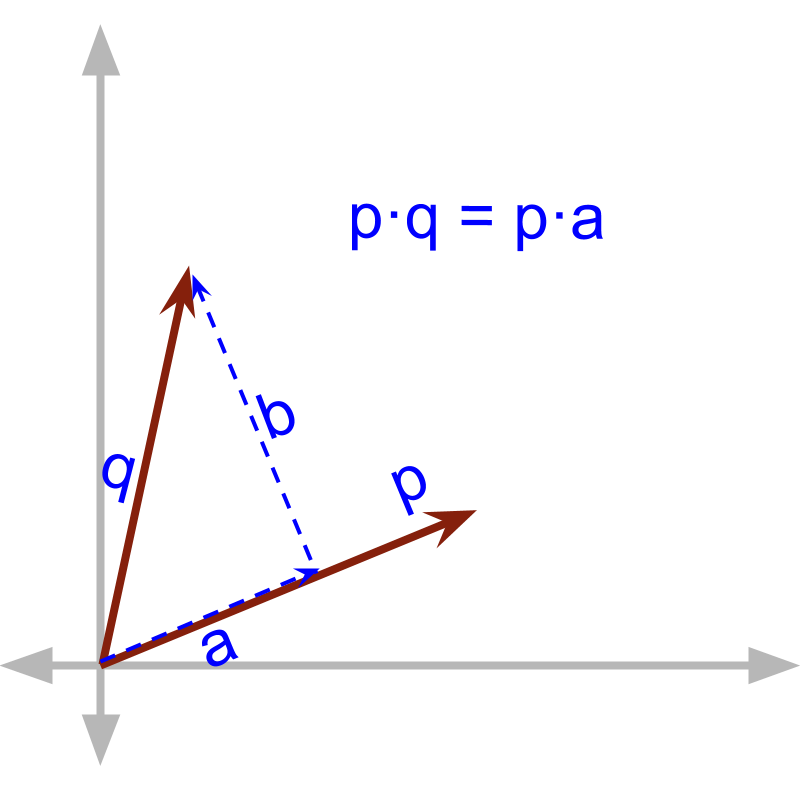→  $\stackrel{\to }{p}\cdot \stackrel{\to }{q}$$\vec{p} \cdot \vec{q}$ $=\stackrel{\to }{p}\cdot \stackrel{\to }{a}$$= \vec{p} \cdot \vec{a}$ $=|\stackrel{\to }{p}||\stackrel{\to }{a}|$$= | \vec{p} | | \vec{a} |$
→  product of magnitudes of components in parallel

maths > vector-algebra > dot-product-projection-form

Vector Dot Product : Projection of a vector

»  component in parallel is calculated by the angle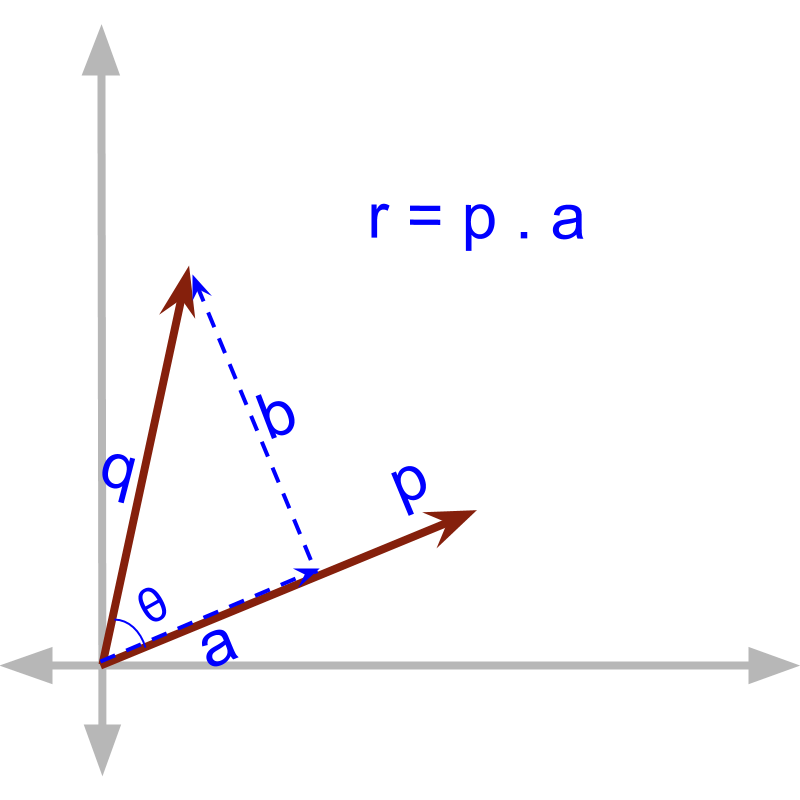→  $\stackrel{\to }{p}\cdot \stackrel{\to }{q}$$\vec{p} \cdot \vec{q}$ $=|\stackrel{\to }{p}||\stackrel{\to }{q}|\mathrm{cos}\theta$$= | \vec{p} | | \vec{q} | \cos \theta$
→  Note that $|\stackrel{\to }{a}|=|\stackrel{\to }{q}|\mathrm{cos}\theta$$| \vec{a} | = | \vec{q} | \cos \theta$
→  $\stackrel{\to }{a}$$\vec{a}$ is the projection of $\stackrel{\to }{q}$$\vec{q}$ on $\stackrel{\to }{p}$$\vec{p}$

maths > vector-algebra > dot-product-component-form

Vector Dot Product : Component Form

»  sum of product of individual components
→  $\stackrel{\to }{p}\cdot \stackrel{\to }{q}= {p}_{x}{q}_{x}+{p}_{y}{q}_{y}+{p}_{z}{q}_{z}$

»  By definition, dot product is scalar

»  Some applications may specify an additional direction in the multiplication of components in parallel. In such cases, the direction is combined.

maths > vector-algebra > introduction-cross-product

Introduction to Cross Product

»  Two products because of orthogonality of components of vectors→  dot product is defined for components in parallel $\stackrel{\to }{p}\cdot \stackrel{\to }{q}$$\vec{p} \cdot \vec{q}$ $=\stackrel{\to }{p}\cdot \stackrel{\to }{a}$$= \vec{p} \cdot \vec{a}$
→  cross product is defined for components in perpendicular $\stackrel{\to }{p}×\stackrel{\to }{q}$$\vec{p} \times \vec{q}$ $=\stackrel{\to }{p}×\stackrel{\to }{b}$$= \vec{p} \times \vec{b}$

maths > vector-algebra > vector-cross-product-first-principles

Cross Product: First Principles

In this page, the vector cross product is explained in first principles.  »  multiplied with component in perpendicular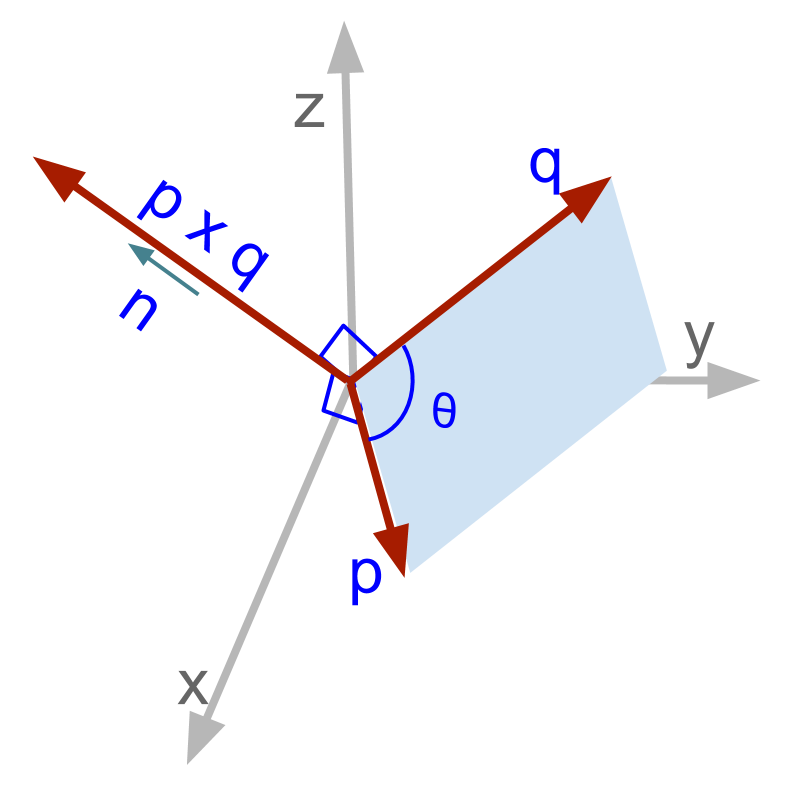→  $\stackrel{\to }{p}×\stackrel{\to }{q}$$\vec{p} \times \vec{q}$ $=\stackrel{\to }{p}×\stackrel{\to }{b}$$= \vec{p} \times \vec{b}$ $=|\stackrel{\to }{p}||\stackrel{\to }{b}|\stackrel{^}{n}$$= | \vec{p} | | \vec{b} | \hat{n}$

→  product of magnitudes of components in perpendicular

→  direction perpendicular to both the vectors

maths > vector-algebra > cross-product-area-of-parallelogram

Cross Product : Area of Parallelogram

»  component in perpendicular is calculated by the angle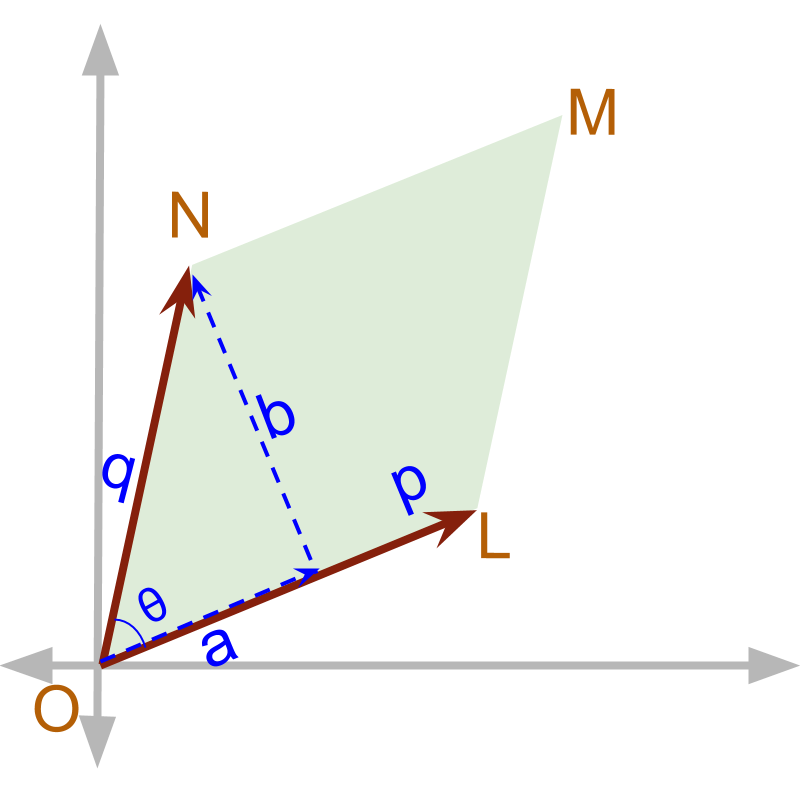→  $\stackrel{\to }{p}×\stackrel{\to }{q}$$\vec{p} \times \vec{q}$ $=|\stackrel{\to }{p}||\stackrel{\to }{q}|\mathrm{sin}\theta \stackrel{^}{n}$$= | \vec{p} | | \vec{q} | \sin \theta \hat{n}$
→  Note that $|\stackrel{\to }{b}|=|\stackrel{\to }{q}|\mathrm{sin}\theta$$| \vec{b} | = | \vec{q} | \sin \theta$
→  $|\stackrel{\to }{p}||\stackrel{\to }{b}|$$| \vec{p} | | \vec{b} |$ is the area of parallelogram with sides $\stackrel{\to }{p}$$\vec{p}$ and $\stackrel{\to }{q}$$\vec{q}$

maths > vector-algebra > vector-cross-product-component-form

Cross Product: Component Form

$\stackrel{\to }{p}×\stackrel{\to }{q}$$\vec{p} \times \vec{q}$
$=|\begin{array}{ccc}i& j& k\\ {p}_{x}& {p}_{y}& {p}_{z}\\ {q}_{x}& {q}_{y}& {q}_{z}\end{array}|$$= | \left(i , j , k\right) , \left({p}_{x} , {p}_{y} , {p}_{z}\right) , \left({q}_{x} , {q}_{y} , {q}_{z}\right) |$
$=\left({p}_{y}{q}_{z}-{p}_{z}{q}_{y}\right)i$$= \left({p}_{y} {q}_{z} - {p}_{z} {q}_{y}\right) i$ $+\left({p}_{z}{q}_{x}-{p}_{x}{q}_{z}\right)j$$+ \left({p}_{z} {q}_{x} - {p}_{x} {q}_{z}\right) j$ $+\left({p}_{x}{q}_{y}-{p}_{y}{q}_{x}\right)k$$+ \left({p}_{x} {q}_{y} - {p}_{y} {q}_{x}\right) k$